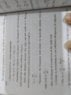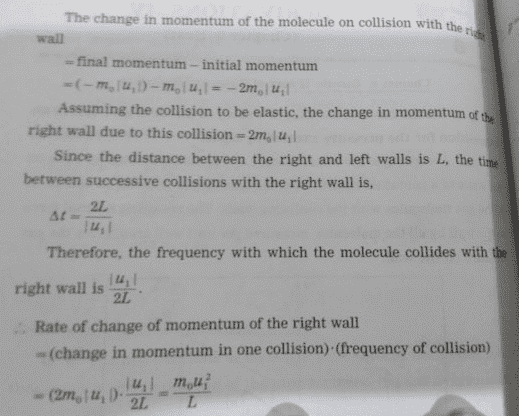# Pressure exerted by a gas (derivation using the kinetic theory of gases)

Bheshaj
In the derivation of finding pressure exerted by a gas using kinetic theory of gases I am not understanding why the time between two collisions is taken as the time for rate of change of momentum when a particle bounces back from the wall. please help me

Homework Helper
I think you misquote. Any reference ? The duration of the collision with the wall is the time in which the momentum changes.

•Bheshaj
Bheshaj
I think you misquote. Any reference ? The duration of the collision with the wall is the time in which the momentum changes.
I think you misquote. Any reference ? The duration of the collision with the wall is the time in which the momentum changes.
see this

#### Attachments

•IMG_20190222_220649.jpg
28.7 KB · Views: 316
Homework HelperSomething gave in in my neck when I tried to read this. Have to go to the hospital first ...

So where is this quote ? I see ##\Delta t = {2L\over |u|} ## for the time to go to the left wall and back again ...

#### Attachments

Homework Helper
They don't have a microscope here in the hospital to read your fine print. Can you be bothered to learn some ##\LaTeX## in order to post legibly ?

Last edited:
Homework Helper
But if I have to guess he now averages the change in momentum from the gas particles that bump into the wall, to calculate the average force on the wall.

Its because yoy want to find average force exerted by the molecule. How u find average force? See u find the time in which this repears aka time period. The collision with wall PQRS repeats. Now find the average force in this time. If u were to find instantaneous force u have to divide by time of collision which is very small.

You are giving Maharashtra HSC? I am also giving it.

•Bheshaj
Bheshaj
Its because yoy want to find average force exerted by the molecule. How u find average force? See u find the time in which this repears aka time period. The collision with wall PQRS repeats. Now find the average force in this time. If u were to find instantaneous force u have to divide by time of collision which is very small.

You are giving Maharashtra HSC? I am also giving it.
yeah, me too thanks

Bheshaj
But if I have to guess he now averages the change in momentum from the gas particles that bump into the wall, to calculate the average force on the wall.
thanks